## Introduction

The scattering of waves through disordered media is a paradigmatic phenomenon that has captured the interest of various communities for quite some time now1, 2, 3. Among the many important physical aspects of wave propagation that have been studied the phenomenon of Anderson localisation has received particular attention4, 5, 6, 7, 8, 9, 10, 11, 12. While much work has been invested into understanding the ‘statistical’ properties of the corresponding wave phenomena13 there has recently been a surge of interest in controlling the scattering of waves through ‘individual’ systems for specific purposes such as detection, imaging, and efficient transmission across disordered materials14, 15. Remarkable progress in these endeavours has recently been made in the optical domain, largely due to the availability of spatial light modulators and new concepts for how to apply them on turbid media16, 17. In a first generation of corresponding experiments the focus was laid on shaping the input wave front impinging on an immutable disordered sample such as to achieve a desired output, like a spatial or temporal focus behind the medium18, 19, 20, 21. More recent studies focused instead on controlling the medium itself, for example, through the material fabrication process22 or through a spatially modulated pumping23, leading, for example, to a versatile control of random and micro-cavity lasers24, 25, 26, 27, 28.

Largely in parallel to these efforts on disordered media, it was recently realised that materials and devices can get entirely new functionalities when adding to them a suitably arranged combination of gain and loss. In particular, structures with a so-called parity time (PT) symmetry29, 30, were recently introduced theoretically31, 32, 33 and experimentally34, 35 in the context of paraxial waveguide optics. On the basis of a delicate balance between gain and loss, these synthetic structures exhibit rich and unconventional behaviour, holding promise for numerous applications in nano photonics and lasers36. In particular, the relation between coherent perfect absorption and scattering through PT-cavities37 as well as unidirectional invisibility in PT-gratings38 have attracted a lot of attention. Symmetry breaking in fibre loop optical networks39, PT-scattering structures40 and periodic PT-systems as new types of metamaterials41 are also active research directions in this new field on PT-optics. Along with these activities, another direction is that of complex lasers that rely on the concepts of PT symmetry breaking and exceptional points. Such synthetic lasers with novel characteristics, are based on loss engineering42. More specifically, coupled PT-symmetric micro disk lasers can lead to optical diodes43, single-mode microring lasers44, synthetic PT-lasers45, loss-induced lasing46, 47 and lasers with chiral modes48, 49. More recent developments include large scale exceptional points in multilayer optical geometries50, transient growth in non-normal lossy potentials51, modulation instabilities in non-Hermitian structures52, non-Hermitian phase matching in optical parametric oscillators53, higher-order exceptional points54, protocols for asymmetric mode switching based on encircling exceptional points dynamically55, 56, and directional cloaking based on non-Hermitian potentials57.

Here we will build on the advances that were made in both of the above research fields with the aim to combine them in a novel and potentially very useful way. We show that for a general disordered medium, given by a distribution of the real part of the refractive index nR(x), a corresponding distribution of its imaginary part nI(x) can be found, such that a wave propagating through this medium will feature a constant intensity throughout the entire non-uniform scattering landscape. In other words, we demonstrate that adding a judiciously chosen distribution of gain and loss to a disordered medium will make waves lose all of their internal intensity variations such that they propagate through the disorder without any back-reflection.

## Materials and methods

### Scattering states without internal intensity variations

The solution strategy that we explore for this purpose is based on the one-dimensional Helmholtz Equation that describes time-independent scattering of a linearly polarised electric field ψ(x) both in forward and in backward direction,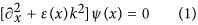Here ɛ(x) is the dielectric permittivity function varying along the spatial coordinate x, k=2π/λ is the wavenumber (with λ being the free space wavelength) and ∂x≡d/dx. The dielectric function is complex thus ɛ(x)=[nR(x)+inI(x)]2. In general, when a plane wave is incident on a spatially varying distribution ɛ(x), interference takes place between the waves propagating forward and backward. As a result, a complex interference pattern is produced with spatial variations on its intensity. As we will now show, this fundamental physical picture can be quite different in the case of non-Hermitian cavities with loss and/or gain.

To jump right to the heart of the matter, we start with an ansatz for a constant-intensity (CI) wave with unit amplitude, ψ(x)=exp[iS(x)], where S(x) is a real valued function. Because of the obvious relation to the semiclassical approximation by Wentzel-Kramers-Brillouin (WKB)58, we will derive the CI-solution of the Helmholtz Equation (1) in the bulk, by demanding that the ansatz ψ(x)=exp[iS(x)] has to be exact in the first order WKB-approximation. As a result of this analysis (see Supplementary Information for details) we obtain the following non-Hermitian dielectric function,that supports a corresponding CI-solution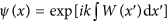at wavenumber k, which solves Equation (1) exactly and is valid for the whole bulk space. At this point we have to emphasise, that the above exact WKB analysis is generally valid (not only in the geometric optics limit). The fact that W(x) can be chosen arbitrarily, with no limitations on its spatial complexity (apart from smoothness), is a key asset of this approach, making it very generally applicable. From this result it is also clear that for vanishing imaginary part [W(x)=const.], the dielectric function as defined in Equation (2), reduces to ɛ(x)=const., resulting in the familiar plane wave in free space. We emphasise that our approach works not only when the functional profile W(x) is known from the outset. Also when a refractive index distribution nR(x) is given, the corresponding gain-loss profile nI(x) can be determined (see Supplementary Information for details).

Furthermore, it can be shown that CI-waves can also be found for all dielectric functions that are described by Equation (2) in a finite domain x[−D, D], bordering on free space for x<−D and x>D. In this case, the scalar Helmholtz-type Equation (1) admits the following exact CI-scattering state: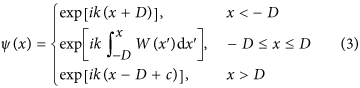where the integration constant c makes sure that the field continuity relations59 are satisfied and is given by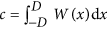. We note at this point that the wavenumber k appearing in Equation (2) is the same as the wavenumber k in the above CI-wave solution. In other words, for any value of k for which a CI-scattering state is desired, the dielectric function ɛ(x) has to be engineered correspondingly. Once ɛ(x) is fixed and plane waves with varying values of k are impinging on this dielectric structure, a perfectly transmitting CI-solution in general only occurs at the predetermined k value inherent in the design of ɛ(x). Due to this restriction to a single frequency also no issues arise with the Kramers-Kronig relations.

Most importantly, the solution in Equation (3) does not only feature a constant intensity |ψ(x)|2=1 in the asymptotic regions x<–D and x>D, where ɛ(x)=1 and simple plane wave propagation is realised, but also inside the finite region of length 2D in which the dielectric function varies and the phase-evolution is non-trivial. Regarding the appropriate boundary conditions at xD, it can be shown that the following perfect transmission boundary conditions (zero reflection)40,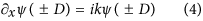imply the following conditions for the generating function,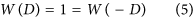We also emphasise that a CI-wave is associated with a specific incidence direction (incidence is assumed here from the left, x<−D, in positive x-direction). When injecting a plane wave with the same predetermined wavenumber k from the other incidence direction (that is, from the right, x>D, in negative x-direction) one still gets perfect transmission simply due to Lorentz-reciprocity. In addition, however, one will also get finite reflection and non-trivial intensity variations inside the potential (|x|≤D).

### Non-Hermitian scattering methods

To elucidate the above ideas, we consider in the section ‘Results and Discussions’ several specific examples of index distributions and study the CI-waves they give rise to. For these calculations, a transfer matrix method59 was used for TE-linearly polarised optical waves along with an effective Hamiltonian approach40. More specifically, the transfer matrix method is valid for piecewise refractive index distributions. To apply such an approach to our scattering problem, we discretised the continuous potential into many slabs of almost constant refractive index, and then applied the transfer matrix method. Another technique we used was that of the effective Hamiltonian. The wave Equation (1),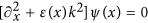, can be written as a generalised eigenvalue problem for the potential of Equation (2) for a given W(x). More specifically we have: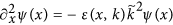. This generalised eigenvalue problem is non-Hermitian due to the perfect transmission boundary conditions. Notice that only one of its eigenmodes will be the CI-state and satisfy the relation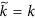. We have compared the two different approaches for specific optical structures and they give identical results.

## Results and discussion

As a first example, we assume W(x) to be a parabolic function modulated with a cosine, namely W(x)=[1−0.2 cos(15πx/2)](2−x2). The corresponding real part of the refractive index distribution nR(x) is shown as the grey shaded area in Figure 1. A wave impinging on this dielectric structure composed of only nR(x) is partly reflected and features a highly oscillatory profile, see Figure 1a. Quite in contrast, when adding also the gain and loss inherent in the imaginary index component nI(x) derived from W(x) (see green and red regions in Figure 1b), the resulting scattering state is fully transmitted and features a constant intensity. Because of the boundary conditions, W(x) must be symmetric at the end points of the cavity, resulting in an anti-symmetric distribution of nI(x). Our example shows that for a plane wave at an arbitrary incident wavenumber k, we can find the corresponding gain-loss landscape (from Equation (2)), such that this wave will fully penetrate the scattering medium without forming any spatial variations in its intensity pattern.

### Perfect transmission through disorder

The most striking application of CI-waves is realised for the case of disordered environments, which is also the focus of our work. We know, for example, that in strongly scattering disordered media Anderson localisation occurs, resulting in an exponential decrease of the transmittance T=|t|2 for structures with sizes greater than the localisation length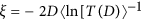. For a given real and disordered index of refraction in the localised regime close to unit transmittance is thus very unlikely and occurs only at well-isolated, sharply resonant wavenumbers that are difficult to achieve experimentally60, 61. Our approach now allows to turn this behaviour upside down—not only in the sense that we can engineer unit transmission at any predetermined value of the wavenumber k but also that we can create scattering states that have constant intensity in a strongly disordered environment which would usually give rise to the most dramatic intensity fluctuations known in wave physics.

We illustrate our results for the disordered one-dimensional slab shown in Figure 2, where a refractive index distribution following Equation (2) is considered with a tunable imaginary component, ɛ(x)=[nR(x)+ianI(x)]2 (the tunable parameter a controls the overall amplitude of gain and loss). From Equation (2), one can understand that CI-scattering-states exist only for a=1. In particular, we choose the generating function W(x) to be a superposition of N Gaussian functions of the same width d, but centred around random positions cn and having random amplitudes rn. More specifically, we consider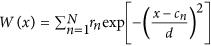, where cn and rn are random variables. This leads us to the following analytical expression of a CI-state ψ(x) in a disordered medium: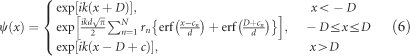where the error function is defined as follows: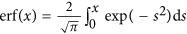, and the constant c is defined as in Equation (3). For a=0 the refractive index is Hermitian, whereas for a=1 CI-waves exist. The refractive index distribution of such a non-Hermitian disordered medium is depicted in Figure 2a, and the localisation length ξ of the Hermitian refractive index (a=0) is depicted in Figure 2b. The imaginary part (gain and loss) of the refractive index distribution that leads (based on Equation (2)) to a CI-state is depicted in Figure 2c. As is physically expected, without the gain and loss distribution, the system reflects almost all waves due to localisation. Furthermore, adding first only the gain part of the refractive index distribution that leads to a CI-state (see Figure 2c) still results in highly oscillatory scattering wave functions with finite reflectance for all values of the gain-loss amplitude a (from 0 to 1), as is illustrated in Figure 2d. Quite counterintuitively, adding also the loss part of the refractive index distribution leads to perfect transmission without any intensity variations for a=1, as demonstrated in Figure 2e. By varying the gain-loss amplitude a, as in Figure 2e, we can thus see a smooth transition from the Anderson localisation regime (at a=0) to perfect transmission with constant intensity (at a=1). Figure 2f shows this transition for the transmittance T(a), indicating the robustness of our approach as well as the fact that already smaller values of a<1 lead to a transmittance that is several orders of magnitude larger than in the absence of gain and loss (T≈2 × 10−3 for a=0). We thus find that the presence of gain and loss in a scattering environment can completely suppress the localisation of waves due to multiple scattering, leading to a delocalised state with a constant intensity and perfect transmission.

A crucial question is the physical values of gain and loss required to observe and realise the proposed CI-waves. It turns out that these values depend directly on the slope and amplitude of the refractive index distribution, as well as on the wavelength of operation, as we can see from Equation (2). The maximum value of gain/loss for the system shown in Figure 2 is at max(nI) ≈ 6.7 × 10−3 with the length of the disorder region being 6 cm. With a wavelength of λ=0.58 μm this would correspond (for a variation of ΔnR ≈ 2 × 10−2) to a gain value of ~1450 cm−1. Typical example gain media at this wavelength that are also implementable in a practical experimental setting are, for example, Rhodamine (6 G) dye materials, commonly used in active plasmonics62 and in opto-fluidic random lasers24. The above gain values are, however, quite high for organic media such that in an experimental implementation smoother refractive index distributions, nR(x), are more realistic, for which the corresponding gain values are smaller. Since, in turn, the localisation length ξ will then be larger (in the absence of gain and loss), also longer disorder regions will be required to see the transition from localised states to CI-waves. We emphasize, however, that our approach is completely scalable also to other wavelengths and gain media. First practical applications that we anticipate will probably also be focused on exploring only a subpart of the above crossover.

### Discrete disordered systems

Certainly the most challenging aspect of CI-waves in terms of their experimental realisation is the fabrication of a specific index distribution with gain and loss63. To overcome such inherent difficulties, we study here also the existence of CI-waves in a system of discrete scatterers, like the one presented in Figure 3. Such a setup is composed of many discrete elements (cavities) with gain or loss and a specific real refractive index distribution. The analytic solution of Equation (2) is still valid in the discrete version of the Helmholtz-type wave Equation: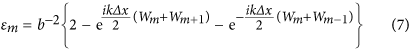and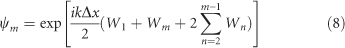where ɛm is the permittivity of the mth scatterer, b=ωΔx, ω2=2[1−cos(kΔx)]/Δx2 and m=1,…, M. In addition, the perfect transmission boundary conditions imposed at the end points of the discrete chain of scatterers ψ0=ψ1 exp(−ikΔx), and ψM+1=ψM exp(ikΔx) as well as the relation ωΔx<2 must always hold. We consider a specific example in Figure 3 of M elements that form a one-dimensional disordered chain. By adding gain or loss onto the sites as prescribed by Equation (7) an incoming wave from the left will have the same constant intensity on all of these sites.

### PT symmetry and mean reality condition

So far we have not discussed the relation of the non-Hermitian distribution (Equation (2)) that supports CI-waves with PT symmetry. For the special case that the generating function is even with respect to x, namely W(x)=W(−x), the dielectric function turns out to be PT-symmetric since ɛ(x)=ɛ*(−x). In other words, our approach is rather general and the only restrictions are the permittivity distribution (Equation (2)) and the boundary conditions (Equation (5)) for W. Keep in mind that Re[ɛ(x)] could in principle also be negative—at least there is no restriction from the mathematical point of view. Since we wish to study relevant physical materials that are easily accessible also experimentally, we choose our W(x) such that we have Re[ɛ(x)]>1 and also nR(x)>1. A direct consequence of these two restrictions is that the spatial average gain-loss over the scattering region is zero,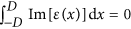. In terms of the refractive index, the evaluation of the integral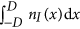depends on the symmetry of W(x). For example, if W(x) is an even function of x, then the refractive index is PT-symmetric, and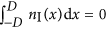. It would be interesting to explore if this condition of ‘mean reality’ also has other interesting consequences that go beyond the restrictions imposed by PT symmetry.

### Effect of wavenumber detuning and gain saturation

As we have already shown analytically and numerically, for a fixed refractive index determined by Equation (2), a CI-wave at the corresponding wavenumber k0 exists. A natural question one may ask at this point is what happens to incident plane waves with detuned wavenumbers k0±Δk0 (Figure 4; when considering active materials that are characterised by approximately flat dispersion curves near the values of the wavelength of operation). Naively, one may expect that the emergence of CI-waves is a sharp resonance phenomenon, so that waves with a slight detuning in the wavenumber k0 should show a completely different behaviour as, for example, around a resonance in a Fabry-Pérot interferometer59. This picture turns out to be misleading on several levels. Since the CI-wave function at position x,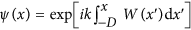, only depends on the generating function W(x') evaluated at values x'<x, one can easily truncate the system at any point x and still get a CI-wave provided one continues the system for all x>x' with a constant generating function that has the same value as at the point of truncation. This behaviour indicates that a refractive index profile that supports CI-waves is not only reflectionless in total but also unidirectional at any point inside a given structure. Perfect transmission in such systems is thus not a resonance phenomenon (as resulting from a back and forth propagation of waves), suggesting that CI-waves are stable against changes of the incident wavelength. To check this explicitly, we numerically calculated the average resonance width of the transmission spectrum |t(k)| of the Hermitian system in Figure 1,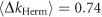, in an interval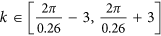, with minimum transmission |t(k)min|=0.94, as is shown in Figure 4. The transmission of waves through the corresponding non-Hermitian CI-refractive index (that of Figure 1b) stays larger than 0.99 over the entire k-interval, confirming our prediction. Another important point to make is that one can easily achieve a transmission equal to one in a non-Hermitian system just by adding enough gain to it. In the scattering landscapes that we consider here, however, the net average amplification is zero, sinceand the intensity is equally distributed everywhere.